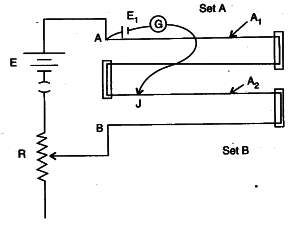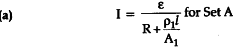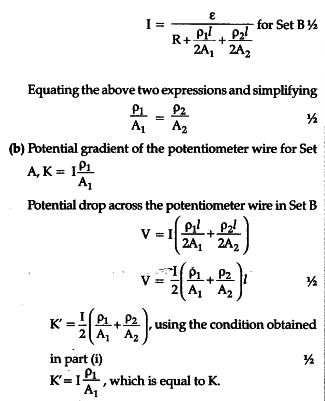# You are given two sets of potentiometer circuit to measure the emf of a cell

You are given two sets of potentiometer circuit to measure the emf of a cell.

Set A : consists of a potentiometer wire of a material of resistivity area of cross-section At and length l.
Set B: consists of a potentiometer of two composite wires of equal lengths 1/2 each, of resistivity and area of cross-section respectively.
(i) Find the relation between resistivity of the two wires with respect to their area of cross section, if the current flowing in the two sets is same.
(ii) Compare the balancing length obtained in the
two sets.Therefore, balancing length obtained in the two sets is same.